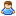1.You are currently browsing our forum as a guest. Create your own forum account to access all forum functionality.

# How do you determine speed in a given direction?

Discussion in 'Programming (In-game)' started by DoktorDoraro, Mar 9, 2019.

This last post in this thread was made more than 31 days old.
1. ### DoktorDoraroTrainee Engineer

Messages:
8
I have a script and I would like to determine the speed of the ship in the forward direction of the ship only. Basically, if you where traveling 10m/s forward and you turned exactly 90 degrees I would get the speed of 0.
--- Automerge ---
After a little bit more digging I found some old code. I updated it and it works great.
Code:
```		/*
* Returns the speed given a cockpit and a direction
* Example direction: Base6Directions.Direction.Forward
*/
public double getShipForwardSpeed(IMyCockpit cockpit, Base6Directions.Direction direction)
{
var forward3I = cockpit.Position + Base6Directions.GetIntVector(cockpit.Orientation.TransformDirection(direction));
var forward = Vector3D.Normalize(Vector3D.Subtract(cockpit.CubeGrid.GridIntegerToWorld(forward3I), cockpit.GetPosition()));

return Vector3D.Dot(cockpit.GetShipVelocities().LinearVelocity, forward);
}```

2. I havent coded in SE for quite a while now but you should be able to use
Code:
```Vector3D Transform(Vector3D position, MatrixD matrix)
```
where the "position" Vector3D is your velocity and the "matrix" Matriis the inverse of your orientation matrix.

That should be far less math than the one you used in the example atm.
It also offers you a good approach to get the other velocity components in local-space so you know the up and right direction components as well.

Code:
```// Your given velocity in world-space
Vector3D velocity; // i.e. someShipController.GetShipVelocities().LinearVelocity;

MatrixD mat; // i.e. someShipController.WorldMatrix.GetOrientation();

// Now we do the actual work
// We transform the world-space velocity into local-space relative to the reference, i.e. the same block we got the velocity from
// Be careful here! We can only just transpose the matrix "mat" because it is an orientation matrix and thus an orthogonal matrix, otherwise we had to "invert" it, which is far more costly
Vector3D localVelocity = Vector3D.Transform(velocity, MatrixD.Transpose(mat));

// Now you can get the length of the -z component of that vector as your forward component. +x is right, +y is up and +z is backwards.
double forwardSpeed = -localVelocity.Z;

```

A simple vector projection should work too, as you dont want that many more informations about the components of the velocity in other directions.

Code:
```// Your given velocity in world-space
Vector3D velocity; // i.e. someShipController.GetShipVelocities().LinearVelocity;

MatrixD mat; // i.e. someShipController.WorldMatrix; // We dont need the orientation now (having a position component is fine)

// Lets project the velocity vector onto the forward direction vector and take its length
double forwardSpeed = velocity.Project(mat.Forward).Length();
```
Note that i did not test any of the two. Thus there could be typing errors as well as something else wrong. Just give it a try if you want3. ### DoktorDoraroTrainee Engineer

Messages:
8
@plaYer2k Thanks for the help! I wasn't able to find project but I found that dot worked fine.
Code:
```		public double getShipDirectionalSpeed(IMyCockpit cockpit, Base6Directions.Direction direction)
{
//get the velocity of the ship as a vector
Vector3D velocity = cockpit.GetShipVelocities().LinearVelocity;

//given a direction calculate the "length" for that direction, lenght is the speed in this case
return velocity.Dot(cockpit.WorldMatrix.GetDirectionVector(direction));
}```
This makes more sense too, as all your basically doing is finding your speed and the direction of your ship/grid and doing some vector math to find the length which in our case is the speed.

I suck at vector math.

•Like x 1